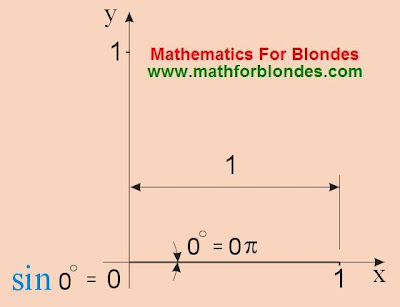10.14.2010

A sine is 0 degrees, sin 0

The sine of zero of degrees equals a zero. On the picture of sine it looks so:Will you, certainly, ask: "And where, actually, sine on this picture"? And there is not he, he in a tiny hole from a zero hid. As a baby mouse in a burrow. To see a baby mouse, it is needed to trick out of him from a burrow. In the animated cartoons assert, the smell of cheese very helps on this business. Sine will not beckon cheese. But there is one piece which on a sine operates smoothly. This magic lure is named for sines is a corner. Not that which babies are put in, and that which in degrees or radians is measured. Let more attentive on we watch this hunt on sines.

We do not have a corner (a corner equals a zero) - there is not a sine.

sin 0° = sin = 0

Now we will try to beckon a sine the littlest value of corner. We will look, as a sine will react on a corner in the zero of degrees, zero of minutes, one thousandth seconds:

sin (0° 0' 0,001") = 0,00000000484813681109

Do you see, the tag of curious spout appeared from a burrow? We will try to increase tenfold our lure and we will take a corner in one hundredth seconds.

sin (0° 0' 0,01") = 0,00000004848136811095

The amount of zeros before numbers grew short on one, and five appeared in the end. Certainly, in a burrow hidden yet very much цифер, which at a desire can be seen. It rather is a boa with a long tail from numbers. Yet tenfold we will increase a corner.

sin (0° 0' 0,1") = 0,00000048481368110954

Did you notice that numbers not nearly changed after zeros? It does not mean that a sine, as well as corner, increases exactly tenfold. Somewhere there, in distance from a comma, numbers change - a baby mouse moves a tail, but we it do not see. We watch only the first twenty numbers after a comma.

Here now for us unique possibility to admire the sine of one second in all his beauty (more precisely, his first two ten of numbers) appeared:

sin (0° 0' 1") = 0,00000484813681107637

We will look farther, as the first ten numbers change after a comma for 10, 20, 30, 40 and 50 seconds (quite naturally, that a superfluous tail we round off) :

sin (0° 0' 10") = 0,0000484814
sin (0° 0' 20") = 0,0000969627
sin (0° 0' 30") = 0,0001454441
sin (0° 0' 40") = 0,0001939255
sin (0° 0' 50") = 0,0002424068

It is possible to consider that for the sake of one minute a sine already fully abandons the burrow and begins quickly to hurry round us. You only will look at a sine 10, 20, 30, 40 and 50 minutes:

sin (0° 1') = 0,0002908882
sin (0° 10') = 0,0029088780
sin (0° 20') = 0,0058177314
sin (0° 30') = 0,0087265355
sin (0° 40') = 0,0116352658
sin (0° 50') = 0,0145438977

I hope, you understand now, that when a corner arrives at all one degree, a sine becomes quite great. A little baby mouse grows into an adult mouse. Look, as quickly the sizes of sine change for corners in 1, 2, 3, 4, 5, 6, 7, 8, 9 and 10 degrees:

sin 1° = 0,017452
sin 2° = 0,034899
sin 3° = 0,052336
sin 4° = 0,069756
sin 5° = 0,087156
sin 6° = 0,104528
sin 7° = 0,121869
sin 8° = 0,139173
sin 9° = 0,156434
sin 10° = 0,173648

Some values of sine of corner alpha on the requests of visitors:

sin 17° = 0,292372

If you watching was not yet tired of a sine, then I suggest to pass to the page a sine 30 degrees. He can be not only seen there but also touch hands, at a desire.